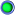View Single Post
oggers

Join Date: Nov 2005
Location: Zulu Time Zone
Posts: 650
As speed of sound is proportional to √absolute temp, it follows that:

The new TAS = 500 x √((50 + 273)/(10 + 273))
= 500 x √(323/283)
= 500 x √1.141
= 500 x 1.07
= 534
Same as BEagle got